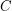### Re: It is proved

Thanks for the reference. The proof, however, seems flawed. The basic outline of the proof (if I understood it correctly) is as follows:

\item Ifhas a sink, then this sink satisfies the conditions. \item An oriented graph without directed cycles has a sink. \item Then one proves directly that a graph containing a directed cycle has a vertex (even on that cycle) that satisfies the conditions.

The last part (Lemma 2 of the manuscript), is not true -- take a directed cycle, add many independent points, and add all the arcs fromto. Now the conjecture is true for this graph (one can take any of the sinks -- vertices in), but it is not true that one can choose a vertex of.

The omission in the proof of Lemma 2 is that it's tacitly assumed, that adjacent vertices of the cycle have no common out-neighbours.